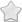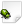CLOSED  Crash report #716  -  [599CF7EC] audio::OpenALSource::setPosition (), r = -nan(0x7fffff), s = -nan(0x7fffff)}, {y = -nan(0x7fffff), g = -nan(0x7fffff), t = -nan(0x7fffff)}, {z = -nan(0x7fffff), b = -nan(0x7fffff), p = -nan(0x7fffff)}}) OpenALSource.cpp:426
Posted Feb 15, 2015 - updated Nov 28, 2015   Shortlink: http://arx.vg/716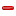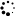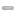0 Votes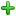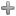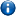This issue is a duplicate of issue #691 - "channel.position contains NAN"This issue has been closed with status "Not a bug" and resolution "DUPLICATE".
Issue details
• Type of issue
Crash report
• Status
 Not a bug
• Assigned to
Not assigned to anyone
• Type of bug
Not triaged
• Likelihood
Not triaged
• Effect
Not triaged
• Posted byDaniel Scharrer
• Owned by
Not owned by anyone
• Estimated time
Not estimated
• Category
Not determined
• Resolution
DUPLICATE
• Priority
Not determined
• Reproducability
Not determined
• Severity
Not determined
• Targetted for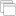Not determined
• OS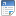Linux
• Architectureamd64
• Fixed inNot determined
Issue description
Illegal instruction: illegal operand

GDB stack trace:
1. [New LWP 14866]
2. [New LWP 13531]
3. [New LWP 13530]
4. [New LWP 13516]
7. 0x00000030b1c10189 in __libc_waitpid (pid=-1, stat_loc=0x7fff4201dc80, options=0) at ../sysdeps/unix/sysv/linux/waitpid.c:40
9. Id Target Id Frame
11. 4 Thread 0x7f319770d700 (LWP 13530) "arx" 0x00000030b14dea1d in poll () at ../sysdeps/unix/syscall-template.S:81
13. 2 Thread 0x7f3194f6d700 (LWP 14866) "Pathfinder" 0x00000030b1c0fe1d in nanosleep () at ../sysdeps/unix/syscall-template.S:81
14. * 1 Thread 0x7f319b7e1740 (LWP 13515) "arx" 0x00000030b1c10189 in __libc_waitpid (pid=-1, stat_loc=0x7fff4201dc80, options=0) at ../sysdeps/unix/sysv/linux/waitpid.c:40
15.
18. No locals.
19. #1 0x00007f319b36f8a3 in cnd_wait (mtx=0x265e098, cond=0x265e0c0) at ../../../../../include/c11/threads_posix.h:154
20. No locals.
21. #2 pipe_semaphore_wait (sema=0x265e098) at ../../../../../src/gallium/auxiliary/os/os_thread.h:248
22. No locals.
24. ws = 0x265dd80
25. cs = <optimized out>
26. i = <optimized out>
27. #4 0x00007f319b36f0d7 in impl_thrd_routine (p=<optimized out>) at ../../../../../include/c11/threads_posix.h:87
28. pack = {func = 0x7f319b36f780 <radeon_drm_cs_emit_ioctl>, arg = 0x265dd80}
30. __res = <optimized out>
31. pd = 0x7f3198832700
32. now = <optimized out>
33. unwind_buf = {cancel_jmp_buf = {{jmp_buf = {139850988857088, 9187815700844570042, 0, 209126039552, 140734300812988, 139850988857088, -9087528795386616390, 9214591553614833082}, mask_was_saved = 0}}, priv = {pad = {0x0, 0x0, 0x0, 0x0}, data = {prev = 0x0, cleanup = 0x0, canceltype = 0}}}
34. not_first_call = <optimized out>
35. pagesize_m1 = <optimized out>
36. sp = <optimized out>
37. freesize = <optimized out>
39. #6 0x00000030b14e7b0d in clone () at ../sysdeps/unix/sysv/linux/x86_64/clone.S:109
40. No locals.
41.
43. #0 0x00000030b14dea1d in poll () at ../sysdeps/unix/syscall-template.S:81
44. No locals.
45. #1 0x00000030c764c986 in poll (__timeout=1000, __nfds=1, __fds=0x7f319770ce10) at /usr/include/bits/poll2.h:46
46. No locals.
47. #2 snd1_pcm_wait_nocheck (pcm=0x27364c0, timeout=1000) at /var/tmp/portage/media-libs/alsa-lib-1.0.28/work/alsa-lib-1.0.28/src/pcm/pcm.c:2418
48. pfd = 0x7f319770ce10
49. revents = 0
50. err = <optimized out>
51. __FUNCTION__ = "snd1_pcm_wait_nocheck"
52. #3 0x00000030b642f588 in ALSAProc (ptr=0x2700900) at /var/tmp/portage/media-libs/openal-1.15.1-r2/work/openal-soft-1.15.1/Alc/backends/alsa.c:507
53. state = 3
54. Device = 0x2700900
55. data = 0x2643170
56. areas = 0x27e3b80
57. update_size = 1024
59. avail = <optimized out>
60. commitres = <optimized out>
61. offset = 0
62. frames = 1024
63. WritePtr = <optimized out>
64. err = <optimized out>
65. __FUNCTION__ = "ALSAProc"
66. #4 0x00000030b64274aa in StarterFunc (ptr=0x278de40) at /var/tmp/portage/media-libs/openal-1.15.1-r2/work/openal-soft-1.15.1/Alc/alcThread.c:96
67. inf = 0x278de40
69. __res = <optimized out>
70. pd = 0x7f319770d700
71. now = <optimized out>
72. unwind_buf = {cancel_jmp_buf = {{jmp_buf = {139850970879744, 9187815700844570042, 0, 209126039552, 5126, 139850970879744, -9087556705694718534, 9214591553614833082}, mask_was_saved = 0}}, priv = {pad = {0x0, 0x0, 0x0, 0x0}, data = {prev = 0x0, cleanup = 0x0, canceltype = 0}}}
73. not_first_call = <optimized out>
74. pagesize_m1 = <optimized out>
75. sp = <optimized out>
76. freesize = <optimized out>
78. #6 0x00000030b14e7b0d in clone () at ../sysdeps/unix/sysv/linux/x86_64/clone.S:109
79. No locals.
80.
83. No locals.
84. #1 0x00000000007dee23 in Lock::lock (this=0x2736860) at /home/dscharrer/pro/arx/src/platform/Lock.cpp:40
85. rc = <optimized out>
86. #2 0x000000000048de55 in Autolock (_lock=<optimized out>, this=<optimized out>) at /home/dscharrer/pro/arx/src/platform/Lock.h:66
87. No locals.
88. #3 audio::update () at /home/dscharrer/pro/arx/src/audio/Audio.cpp:227
89. No locals.
90. #4 0x00000000006a1e5f in SoundUpdateThread::run (this=0x26437a0) at /home/dscharrer/pro/arx/src/scene/GameSound.cpp:1707
91. No locals.
95. __res = <optimized out>
96. pd = 0x7f319760c700
97. now = <optimized out>
98. unwind_buf = {cancel_jmp_buf = {{jmp_buf = {139850969827072, 9187815700844570042, 0, 209126039552, 140734300818504, 139850969827072, -9087556572013861446, 9214591553614833082}, mask_was_saved = 0}}, priv = {pad = {0x0, 0x0, 0x0, 0x0}, data = {prev = 0x0, cleanup = 0x0, canceltype = 0}}}
99. not_first_call = <optimized out>
100. pagesize_m1 = <optimized out>
101. sp = <optimized out>
102. freesize = <optimized out>
104. #7 0x00000030b14e7b0d in clone () at ../sysdeps/unix/sysv/linux/x86_64/clone.S:109
105. No locals.
106.
108. #0 0x00000030b1c0fe1d in nanosleep () at ../sysdeps/unix/syscall-template.S:81
109. No locals.
111. t = {tv_sec = 0, tv_nsec = 10000000}
112. #2 0x0000000000443d3f in PathFinderThread::run (this=0x3004570) at /home/dscharrer/pro/arx/src/ai/PathFinderManager.cpp:324
113. curpr = <optimized out>
114. eb = <optimized out>
115. pathfinder = {radius = 25, height = -45, heuristic = 0.25999999, map_s = 5997, map_d = 0x5168c40, slight_c = 1200, slight_l = 0xe3a380 <GLight>}
119. __res = <optimized out>
120. pd = 0x7f3194f6d700
121. now = <optimized out>
122. unwind_buf = {cancel_jmp_buf = {{jmp_buf = {139850929329920, 9187815700844570042, 0, 50515728, 21, 139850929329920, -9087555554643483206, 9214591553614833082}, mask_was_saved = 0}}, priv = {pad = {0x0, 0x0, 0x0, 0x0}, data = {prev = 0x0, cleanup = 0x0, canceltype = 0}}}
123. not_first_call = <optimized out>
124. pagesize_m1 = <optimized out>
125. sp = <optimized out>
126. freesize = <optimized out>
128. #5 0x00000030b14e7b0d in clone () at ../sysdeps/unix/sysv/linux/x86_64/clone.S:109
129. No locals.
130.
132. #0 0x00000030b1c10189 in __libc_waitpid (pid=-1, stat_loc=0x7fff4201dc80, options=0) at ../sysdeps/unix/sysv/linux/waitpid.c:40
133. resultvar = 35412344
134. oldtype = 0
135. #1 0x000000000067cbba in CrashHandlerPOSIX::handleCrash (this=0x21c5400, signal=signal@entry=4, code=code@entry=2) at /home/dscharrer/pro/arx/src/platform/crashhandler/CrashHandlerPOSIX.cpp:227
136. status = 3
137. argument = "[", '\000' <repeats 15 times>, "n\000\000\000\377\177", '\000' <repeats 18 times>, "w\000\000\000|\000\000\000\330\251\246\000\000\000\000\000\000\244\350\251\070<\227\026\005\000\000\000\000\000\000\000@Fy\261\060\000\000\000\032\000\000\000\000\000\000\000}", '\000' <repeats 15 times>, "\240v\345\002\000\000\000\000@\342\001B\377\177\000\000\356\323G\261\060", '\000' <repeats 11 times>, "\032\000\000\000\000\000\000\000@\362\001B\377\177\000\000\230\374\305\262\060\000\000\000\001\000\000\000\000\000\000\000\071x\314\262\060\000\000\000\300\251\246\000\000\000\000\000[\206\314\262\060", '\000' <repeats 11 times>...
138. args = {0x3 <error: Cannot access memory at address 0x3>, 0x7fff4201dd50 "\300\251\246", 0x7fff00000030 <error: Cannot access memory at address 0x7fff00000030>}
139. #2 0x000000000067ccdc in signalHandler (signal=4, info=<optimized out>, context=<optimized out>) at /home/dscharrer/pro/arx/src/platform/crashhandler/CrashHandlerPOSIX.cpp:50
140. No locals.
141. #3 <signal handler called>
142. No locals.
143. #4 0x00000000004a7258 in audio::OpenALSource::setPosition (this=0x50d08a0, position=@0x50d08c0: {{x = -nan(0x7fffff), r = -nan(0x7fffff), s = -nan(0x7fffff)}, {y = -nan(0x7fffff), g = -nan(0x7fffff), t = -nan(0x7fffff)}, {z = -nan(0x7fffff), b = -nan(0x7fffff), p = -nan(0x7fffff)}}) at /home/dscharrer/pro/arx/src/audio/openal/OpenALSource.cpp:426
144. No locals.
145. #5 0x00000000004ad80a in audio::OpenALSource::init (this=this@entry=0x50d08a0, _id=_id@entry=131746, inst=inst@entry=0x0, _channel=@0x7fff4201e8c0: {flags = {flags = 34348}, mixer = {t = 1}, volume = 1, pitch = 1.09537768, pan = 2.14321089e-37, position = {{x = -nan(0x7fffff), r = -nan(0x7fffff), s = -nan(0x7fffff)}, {y = -nan(0x7fffff), g = -nan(0x7fffff), t = -nan(0x7fffff)}, {z = -nan(0x7fffff), b = -nan(0x7fffff), p = -nan(0x7fffff)}}, velocity = {{x = 0, r = 0, s = 0}, {y = 0, g = 0, t = 0}, {z = 0, b = 0, p = 0}}, direction = {{x = 0, r = 0, s = 0}, {y = 0, g = 0, t = 0}, {z = 0, b = 0, p = 0}}, cone = {inner_angle = 0, outer_angle = 0, outer_volume = 32.4852905}, falloff = {start = 200, end = 2200}}) at /home/dscharrer/pro/arx/src/audio/openal/OpenALSource.cpp:226
146. No locals.
147. #6 0x0000000000498dbe in audio::OpenALBackend::createSource (this=0x2494d30, sampleId=<optimized out>, channel=@0x7fff4201e8c0: {flags = {flags = 34348}, mixer = {t = 1}, volume = 1, pitch = 1.09537768, pan = 2.14321089e-37, position = {{x = -nan(0x7fffff), r = -nan(0x7fffff), s = -nan(0x7fffff)}, {y = -nan(0x7fffff), g = -nan(0x7fffff), t = -nan(0x7fffff)}, {z = -nan(0x7fffff), b = -nan(0x7fffff), p = -nan(0x7fffff)}}, velocity = {{x = 0, r = 0, s = 0}, {y = 0, g = 0, t = 0}, {z = 0, b = 0, p = 0}}, direction = {{x = 0, r = 0, s = 0}, {y = 0, g = 0, t = 0}, {z = 0, b = 0, p = 0}}, cone = {inner_angle = 0, outer_angle = 0, outer_volume = 32.4852905}, falloff = {start = 200, end = 2200}}) at /home/dscharrer/pro/arx/src/audio/openal/OpenALBackend.cpp:251
148. orig = 0x0
149. index = 2
150. id = 131746
151. #7 0x00000000004932f0 in audio::samplePlay (sample_id=@0x7fff4201e8bc: -64862, channel=@0x7fff4201e8c0: {flags = {flags = 34348}, mixer = {t = 1}, volume = 1, pitch = 1.09537768, pan = 2.14321089e-37, position = {{x = -nan(0x7fffff), r = -nan(0x7fffff), s = -nan(0x7fffff)}, {y = -nan(0x7fffff), g = -nan(0x7fffff), t = -nan(0x7fffff)}, {z = -nan(0x7fffff), b = -nan(0x7fffff), p = -nan(0x7fffff)}}, velocity = {{x = 0, r = 0, s = 0}, {y = 0, g = 0, t = 0}, {z = 0, b = 0, p = 0}}, direction = {{x = 0, r = 0, s = 0}, {y = 0, g = 0, t = 0}, {z = 0, b = 0, p = 0}}, cone = {inner_angle = 0, outer_angle = 0, outer_volume = 32.4852905}, falloff = {start = 200, end = 2200}}, play_count=1) at /home/dscharrer/pro/arx/src/audio/Audio.cpp:632
152. source = 0x0
153. #8 0x000000000069b1d7 in ARX_SOUND_PlayScript (name=@0x7fff4201ea40: {pathstr = "frog1.wav"}, io=io@entry=0x5292d80, pitch=pitch@entry=1.09537768, loop=loop@entry=ARX_SOUND_PLAY_ONCE) at /home/dscharrer/pro/arx/src/scene/GameSound.cpp:773
154. channel = {flags = {flags = 34348}, mixer = {t = 1}, volume = 1, pitch = 1.09537768, pan = 2.14321089e-37, position = {{x = -nan(0x7fffff), r = -nan(0x7fffff), s = -nan(0x7fffff)}, {y = -nan(0x7fffff), g = -nan(0x7fffff), t = -nan(0x7fffff)}, {z = -nan(0x7fffff), b = -nan(0x7fffff), p = -nan(0x7fffff)}}, velocity = {{x = 0, r = 0, s = 0}, {y = 0, g = 0, t = 0}, {z = 0, b = 0, p = 0}}, direction = {{x = 0, r = 0, s = 0}, {y = 0, g = 0, t = 0}, {z = 0, b = 0, p = 0}}, cone = {inner_angle = 0, outer_angle = 0, outer_volume = 32.4852905}, falloff = {start = 200, end = 2200}}
155. sample_id = -64862
156. #9 0x0000000000704187 in script::(anonymous namespace)::PlayCommand::execute (this=0x291dc00, context=@0x7fff4201f0f0: {script = 0x52936f0, pos = 959, entity = 0x5292d80, message = SM_EXECUTELINE, stack = std::vector of length 0, capacity 0}) at /home/dscharrer/pro/arx/src/script/ScriptedConversation.cpp:169
157. num = <optimized out>
158. loop = ARX_SOUND_PLAY_ONCE
159. stop = <optimized out>
160. no_pos = <optimized out>
161. sample = {pathstr = "frog1.wav"}
162. unique = <optimized out>
163. pitch = 1.09537768
164. options = "-p"
165. #10 0x000000000078f5e1 in ScriptEvent::send (es=0x52936f0, msg=SM_DUMMY, params=<optimized out>, io=0x5292d80, evname="", info=<optimized out>) at /home/dscharrer/pro/arx/src/script/ScriptEvent.cpp:409
166. command = @0x291dc00: {<boost::noncopyable_::noncopyable> = {<No data fields>}, _vptr.Command = 0x8031f0 <vtable for script::(anonymous namespace)::PlayCommand 16>, name = "play", entityFlags = -1}
167. res = <optimized out>
168. word = "play"
169. it = {first = "play", second = }
170. brackets = 18446744073709551615
171. ret = <optimized out>
172. eventname = ""
173. esss = <optimized out>
174. context = {script = 0x52936f0, pos = 959, entity = 0x5292d80, message = SM_EXECUTELINE, stack = std::vector of length 0, capacity 0}
175. pos = <optimized out>
176. #11 0x00000000006db4f1 in ARX_SCRIPT_Timer_Check () at /home/dscharrer/pro/arx/src/script/Script.cpp:1885
177. es = 0x52936f0
178. st = 0x2926630
179. name = "test"
180. i = 57
181. #12 0x00000000004b98c0 in ArxGame::updateLevel (this=this@entry=0x21c6200) at /home/dscharrer/pro/arx/src/core/ArxGame.cpp:1942
182. No locals.
183. #13 0x00000000004badb0 in ArxGame::render (this=this@entry=0x21c6200) at /home/dscharrer/pro/arx/src/core/ArxGame.cpp:2294
184. No locals.
185. #14 0x00000000004bb069 in ArxGame::doFrame (this=this@entry=0x21c6200) at /home/dscharrer/pro/arx/src/core/ArxGame.cpp:1321
186. No locals.
187. #15 0x00000000004bb74a in ArxGame::run (this=0x21c6200) at /home/dscharrer/pro/arx/src/core/ArxGame.cpp:1250
188. No locals.
189. #16 0x00000000004c958f in runGame () at /home/dscharrer/pro/arx/src/core/Core.cpp:301
190. No locals.
191. #17 0x000000000041a5c1 in main (argc=5, argv=0x7fff42020c48) at /home/dscharrer/pro/arx/src/core/Startup.cpp:178
192. status = RunProgram

Steps to reproduce this issue
nan nan nan nan

reproducible in the goblin kingdom

#2
Comment posted byDaniel Scharrer
Feb 15, 12:26
A file was uploaded. arx.log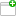This comment was attached:

arxcrash-13515-2133381021
#4
Comment posted byDaniel Scharrer
Feb 15, 12:26
A file was uploaded. cfg.iniThis comment was attached:

arxcrash-13515-2133381021
#5
Comment posted byDaniel Scharrer
Feb 15, 12:26
A file was uploaded. crash.xmlThis comment was attached:

arxcrash-13515-2133381021